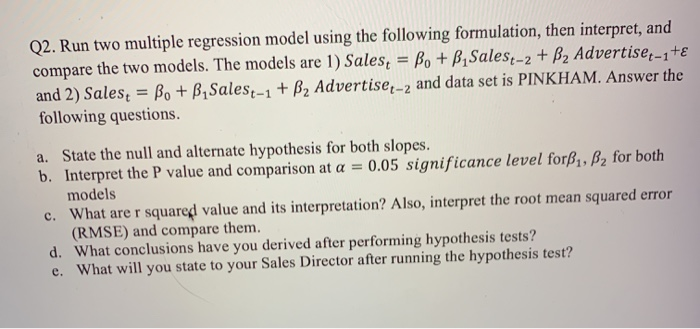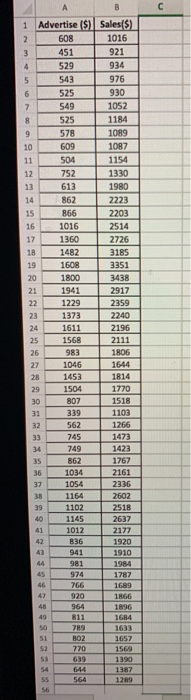Homework Help Question & Answers

# ill leave a like Q2. Run two multiple regression model using the following formulation, then interpret,...ill leave a like
Q2. Run two multiple regression model using the following formulation, then interpret, and compare the two models. The models are 1) Sales, = Be + B Salese-2 + B2 Advertiset-1+€ and 2) Sales; = B. + BSalest-1 + B2 Advertise-2 and data set is PINKHAM. Answer the following questions. a. State the null and alternate hypothesis for both slopes. b. Interpret the P value and comparison at a = 0.05 significance level forB1, B2 for both models c. What are r squared value and its interpretation? Also, interpret the root mean squared error (RMSE) and compare them. d. What conclusions have you derived after performing hypothesis tests? e. What will you state to your Sales Director after running the hypothesis test?
1 Sales(\$) 1016 921 3 1934 Advertise (\$) 608 451 529 543 525 549 525 578 976 930 1052 1184 8 10.89 609 1097 1154 1320 504 752 613 14862 8.66 1980 2223 2203 1016 2514 1360 1482 2726 3185 3351 3438 1608 1800 2917 1229 2359 2240 1373 1611 2196 2111 1568 983 1046 28 1453 29 1504 30807 339 1806 1644 1814 1770 1518 1103 1266 1473 1423 1767 2161 32 562 745 749 862 1034 1054 1164 1102 2602 1012 2177 1920 836 941 1910 766 1699 811

#### Homework Answers

Answer #1

Model 1

Regression Analysis: Sales versus SalesT_2, AdvertiseT_1

Analysis of Variance

 Source DF Adj SS Adj MS F-Value P-Value Regression 2 16450153 8225076 93.49 0.000 SalesT_2 1 1738154 1738154 19.76 0.000 AdvertiseT_1 1 3234076 3234076 36.76 0.000 Error 49 4310891 87977 Total 51 20761043

Model Summary

 S R-sq R-sq(adj) R-sq(pred) 296.610 79.24% 78.39% 76.08%

Coefficients

 Term Coef SE Coef T-Value P-Value VIF Constant 168 133 1.26 0.214 SalesT_2 0.4177 0.0940 4.44 0.000 1.98 AdvertiseT_1 0.951 0.157 6.06 0.000 1.98

Regression Equation

 Sales = 168 + 0.4177 SalesT_2 + 0.951 AdvertiseT_1

Model 2

Regression Analysis: Sales versus SalesT_1, AdvertiseT_2

Analysis of Variance

 Source DF Adj SS Adj MS F-Value P-Value Regression 2 17990758 8995379 159.11 0.000 SalesT_1 1 5656199 5656199 100.05 0.000 AdvertiseT_2 1 5715 5715 0.10 0.752 Error 49 2770285 56536 Total 51 20761043

Model Summary

 S R-sq R-sq(adj) R-sq(pred) 237.774 86.66% 86.11% 84.94%

Coefficients

 Term Coef SE Coef T-Value P-Value VIF Constant 103 104 0.99 0.325 SalesT_1 0.9675 0.0967 10.00 0.000 3.36 AdvertiseT_2 -0.052 0.165 -0.32 0.752 3.36

Regression Equation

 Sales = 103 + 0.9675 SalesT_1 - 0.052 AdvertiseT_2

(a) H0: Beta1 = Beta2 = 0 vs H1: At least one of Beta1 and Beta2 is not zer
(b) For model1 p values for both the parameters are 0.000. That is, both are significant. While for model the p value for beta1 is 0.000 indicating its significance while p value for beta2 is 0.752 implying its insignificance.

(c) Model 1: R square = 0.7924 and RMSE = 2076.27

Model2: R square = 0.8666 and RMSE = 1664.42

For model1 79.24% of the variation in sales is explained by salesT-2 and AdvertiseT-1 while for model 86.66% of the variation in sales is explained by salesT-1 and AdvertiseT-2. This indicates model2 is better fit. The RMSE for model2 is smaller than that of model 1 implying that model 2 is better.

(d) conclusion is written in (b)

(e) As per model1 SalesT-2 and AdvertiseT-1 both the variables can be used to predict the sales and as per model2 SalesT-1 only can be used to predict the sales as AdvertiseT-2 is insignificant. Model2 is better fit than model1 if we compare the R square and RMSE values. Even though model1 is inferior to model2 both the variables in SalesT-2 and AdvertiseT-1 are significant. Hence it is suggested to fit another model with SalesT-1, SalesT-2 and AdvertiseT-1 as the predictors of the sales.

Know the answer?
Your Answer:

#### Post as a guest

Your Name:

What's your source?

#### Earn Coin

Coins can be redeemed for fabulous gifts.

Not the answer you're looking for? Ask your own homework help question. Our experts will answer your question WITHIN MINUTES for Free.
Similar Homework Help Questions
Free Homework App

Scan Your Homework
to Get Instant Free Answers
Need Online Homework Help?

Get Answers For Free
Most questions answered within 3 hours.